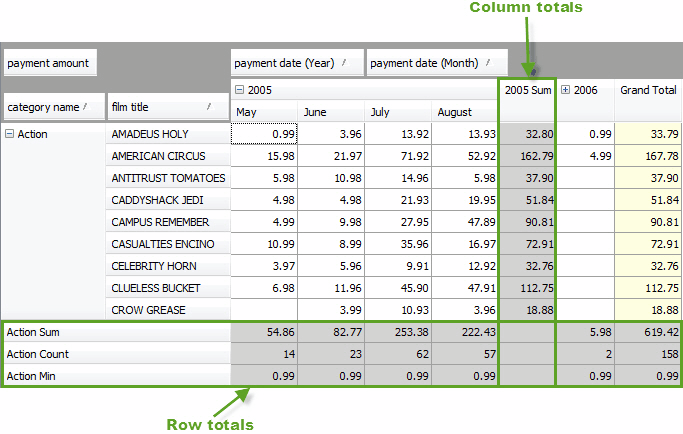# Totals

Row Totals are the rows containing a calculated sum of row field values. Column totals are the columns containing a calculated sum of column field values.

Totals are calculated basing on the selected summary functions and displayed in the Data area highlighted with the grey color. The default function calculates the sum of all values. You can cancel the default function or, quite the contrary, add others.